# Accelerating 16/32-Bit Math Operations with the DS80C390/DS80C400

### Abstract

The Maxim DS80C390/DS80C400 high-speed microcontroller offers the end user a dedicated hardware 16/32-bit math accelerator. Accessing the math accelerator is accomplished by using five dedicated special function registers. 16-bit multiply and 32-bit divide operations are realized with the DS80C390/DS80C400. This application note provides the designer with helpful information about the math acceleration features of the DS80C390/DS80C400 high-speed microcontroller and various code examples.

### Overview

Performance advances in the 8051 microcontroller market have increasingly led users to consider 8-bit devices in applications that once required processing power only available from 16-bit devices. Some of these potential applications, unquestionably, require fast mathematical operations and computations involving 16-bit data. The DS80C390/DS80C400 addresses this need on two levels. This faster instruction execution generates peak performance of 10MIPS and 18.75MIPS respectively for the DS80C390 and DS80C400. This faster instruction execution, coupled with a 40MHz maximum clock frequency, generates a peak performance of 10MIPS. Equally important to applications doing intensive 16-bit math is the inclusion of dedicated hardware for 16/32-bit math acceleration. This application note will explain the interface and operation of the on-chip math accelerator, and give some examples of its use.

### Accessing the Math Accelerator Hardware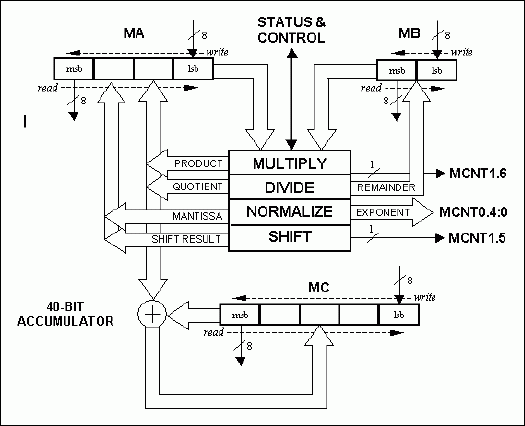SFR (Addr) Bit NAME(S) Accelerator Usage By Operation (MUL, DIV16, DIV32, NORM, SHIFT) OPERATION BEFORE AFTER MCNT0 (D1h) MCNT0.7 Active-low LSHIFT SHIFT 0 = left, 1 = right MCNT0.6 CSE SHIFT 1 = enables circular shift MCNT0.5 SCE SHIFT 1 = include SBC in shift MCNT0.4-0 MAS4:0 SHIFT Number of shifts to do NORM 00000b = start NORM Number of shifts done MCNT1 (D2h) MCNT1.7 MST ALL Busy Bit Busy Bit MCNT1.6 MOF MUL 1 = product > FFFFh DIV 16/32 1 = divide by 0 attempt MCNT1.5 SCB SHIFT Carry Bit Carry Bit MCNT1.4 CLM ALL Clear MA, MB, and MC Clear MA, MB, and MC MA (D3h) MUL 16-bit multiplicand 32-bit product DIV16 16-bit dividend 16-bit quotient DIV32 32-bit dividend 32-bit quotient NORM 32-bit data 32-bit mantissa SHIFT 32-bit data 32-bit shifted result MB (D4h) MUL 16-bit multiplier DIV 16/32 16-bit divisor 16-bit remainder MC (D5h) MUL DIV16 DIV32 40-bit accumulator 40-bit accumulator

### Supported Operations

The DS80C390/DS80C400 math accelerator supports four basic operations: multiply, divide, normalize, and shift. The operation to be performed by the math accelerator is defined by the sequence in which three registers (MA, MB, and MCNT0) are written. The BUSY bit (MCNT1.7) indicates when an operation has started (BUSY = 1) and when the operation completes (BUSY = 0). Each math accelerator operation, however, is guaranteed to complete within a fixed number of machine cycles, eliminating the need for BUSY bit polling. The table below gives the hardware execution time for each accelerator operation. Each math accelerator operation and SFR write sequence needed to initiate the operation is described after the table. This information can also be found in the DS80C390 User's Guide Supplement or High-Speed Microcontroller User's Guide: Network Microcontroller Supplement.

 OPERATION EXECUTION TIME MIN EXECUTION TIME (tCLCL = 25ns; 40MHz) Multiply 16-bit x 16-bit 24 tCLCL 600ns Divide 32-bit/16-bit 36 tCLCL 900ns Divide 16-bit/16-bit 24 tCLCL 600ns Normalize 32-bit 36 tCLCL 900ns Shift 32-bit 36 tCLCL 600ns

### Multiply 16-Bit x 16-Bit

The 16-bit by 16-bit multiply operation is initiated by writing the 16-bit multiplier to the MB register, followed by writing the 16-bit multiplicand to the MA register. Each 16-bit word must be loaded least significant byte first to the required registers. The accelerator hardware completes the multiply operation within six machine cycles producing a 32-bit result which is accessible, most significant byte first, by reading the MA register. Four reads of the MA register are necessary to acquire the entire product. The multiply operation automatically accumulates the product with the previous contents of the 40-bit accumulator and sets the MOF flag (MCNT1.6) if the product exceeds 0000FFFFh.

### Divide 32-Bit/16-Bit and Divide 16-Bit/16-Bit

The 32-bit divide by 16-bit operation is initiated by writing the 32-bit dividend to the MA register, followed by writing the 16-bit divisor to the MB register. The 16-bit divide by 16-bit operation is initiated similarly except that two fewer bytes need be written to the MA register for the 16-bit dividend. All 32-bit double word and 16-bit word data must be loaded least significant byte first to the required registers. The math accelerator completes the 32/16 division within nine machine cycles and the 16/16 division within six machine cycles, generating a 32-bit or 16-bit quotient depending upon the size of the initial dividend. A 16-bit remainder is generated for both divide operations. The quotient and remainder are accessible, most significant byte first, by reading the MA and MB registers respectively. For the 16/16 divide, two reads of the MA register will return the 16-bit quotient while the 32/16 divide requires four reads of MA in order to get the full 32-bit quotient. It does not matter whether the quotient or remainder is read first. The divide operation automatically accumulates the quotient with the previous contents of the 40-bit accumulator and sets the MOF flag (MCNT1.6) if the divisor was 0000h.

### Normalize 32-Bit

The 32-bit normalize operation is initiated by writing a 32-bit double word to MA and then writing the MAS4:0 (MCNT0.4-0) bits = 00000b. The normalization will complete within nine machine cycles. At that time, the left shifted 32-bit result can be read, most significant byte first, from the MA register. The number of left shifts needed to normalize the 32-bit double word will be returned to the MAS4:0 bits.

### Shift Right/Left 32-Bit

The 32-bit shift operation is initiated by writing a 32-bit double word to the MA register, followed by a write to the MAS4:0 bits of the number of shifts to execute. The bit shifting can optionally be done in a right or left direction, be defined as a circular shift, and be inclusive or exclusive of the carry (SCB) bit. When circular shifting is not performed, zero bit data (0) will always be shifted in during the operation. The figure below details the controls for the shift operation.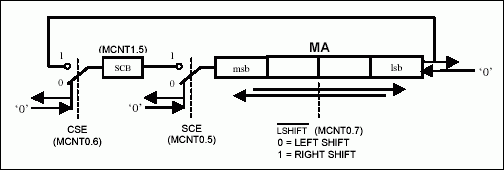### 40-Bit Accumulator

The 40-bit accumulator adds the result of each multiply (product) or divide (quotient) to its current contents. The entire 40-bit accumulator can be loaded, least significant byte first, with five writes to the MC special function register. Similarly, the 40-bit accumulator can be read, most significant byte first, with five reads of the MC special function register.

### Assembly Code Examples of Each Accelerator Operation

Below are simple code examples to demonstrate the required load/unload procedure for each operation and diagrams to highlight the registers and bits involved in each.

### Multiply 16-Bit × 16-Bit

```mov mb, #78h ; lsb (5678h)
mov mb, #56h ; msb (5678h)
mov ma, #34h ; lsb (1234h)
mov ma, #12h ; msb (1234h)
nop ; mb, mb, ma, ma write sequence => 16-bit * 16-bit
nop
nop
nop
nop
nop ; 32-bit product ready after 6 machine cycles
mov r0, ma ; r0 = 06h (msb)
mov r1, ma ; r1 = 26h
mov r2, ma ; r2 = 00h
mov r3, ma ; r3 = 60h (lsb)
```### Divide 32-Bit/16-Bit

```mov ma, #78h ; lsb (56785678h)
mov ma, #56h ; lsb+1 (56785678h)
mov ma, #78h ; lsb+1 (56785678h)
mov ma, #56h ; msb (56785678h)
mov mb, #34h ; lsb (1234h)
mov mb, #12h ; msb (1234h)
nop ; ma, ma, ma, ma, mb, mb write sequence
nop ; => 32-bit/16-bit
nop
nop
nop
nop
nop
nop
nop ; quotient & remainder ready after 9 machine cycles
mov r0, ma ; r0 = 00h (msb quotient)
mov r1, ma ; r1 = 04h
mov r2, ma ; r2 = c0h
mov r3, ma ; r3 = 12h (lsb quotient)
mov r6, mb ; r6 = 0eh (msb remainder)
mov r7, mb ; r7 = d0h (lsb remainder)
```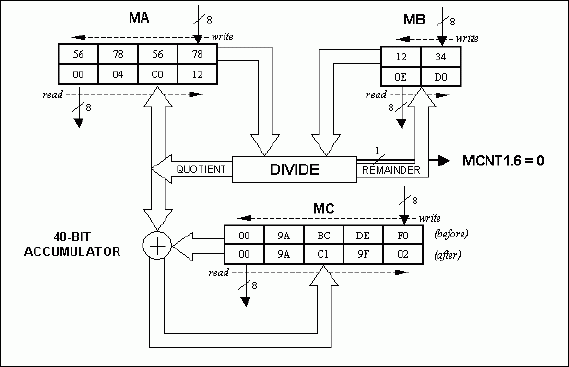### Divide 16-Bit/16-Bit

```mov ma, #78h ; lsb (5678h)
mov ma, #56h ; msb (5678h)
mov mb, #34h ; lsb (1234h)
mov mb, #12h ; msb (1234h)
nop ; ma, ma, mb, mb write sequence => 16-bit/16-bit
nop
nop
nop
nop
nop ; quotient & remainder ready after 6 machine cycles
mov r4, ma ; r4 = 00h (msb quotient)
mov r5, ma ; r5 = 04h (lsb quotient)
mov r6, mb ; r6 = 0dh (msb remainder)
mov r7, mb ; r7 = a8h (lsb remainder)
```### Normalize 32-Bit

```mov ma, #67h ; lsb (01234567h)
mov ma, #45h ; lsb+1 (01234567h)
mov ma, #23h ; lsb+1 (01234567h)
mov ma, #01h ; msb (01234567h)
anl mcnt0, #0e0h ; mas4:0=00000b
nop ; ma, ma, ma, ma, mcnt0.4-0=00000b
nop ; write sequence => 32-bit normalize
nop
nop
nop
nop
nop
nop
nop ; mantissa/exponent ready after 9 machine cycles
mov r0, ma ; r0 = 91h (msb mantissa)
mov r1, ma ; r1 = a2h
mov r2, ma ; r2 = b3h
mov r3, ma ; r3 = 80h (lsb mantissa)
mov a, mcnt0
anl a, #1fh
mov r7, a ; r7 = 07h (#shifts)
```### Shift Right/Left 32-Bit

```orl mcnt1, #20h ; scb=1
mov ma, #80h ; lsb (91a2b380h)
mov ma, #0b3h ; lsb+1 (91a2b380h)
mov ma, #0a2h ; lsb+1 (91a2b380h)
mov ma, #91h ; msb (91a2b380h)
mov mcnt0, #0e7h ; lshift\=1, cse=1, sce=1, mas4:0=7h
nop ; ma, ma, ma, ma, mcnt0.4-0=00111b
nop ; write sequence => 32-bit shift
nop ; circular right shift w/scb
nop
nop
nop
nop
nop
nop ; shifted result ready after 9 machine cycles
mov r0, ma ; r0 = 03h (msb shifted result)
mov r1, ma ; r1 = 23h
mov r2, ma ; r2 = 45h
mov r3, ma ; r3 = 67h (lsb shifted result)
```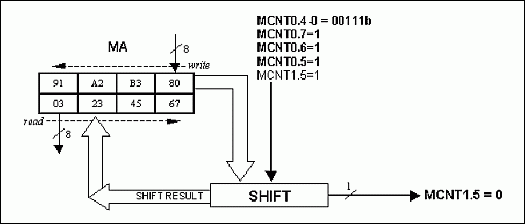### Interrupting Accelerator Operation

As discussed earlier, the math accelerator hardware is controlled entirely by the sequence in which the associated SFRs are written and read. Each register read or write points to a different physical memory location. To achieve correct results, it is critical that the prescribed order not be violated. As a rule, the math accelerator should not be interrupted by another task that also uses the math accelerator, as this will generally produce undesired results. Simply put, there exists only one math accelerator capable of performing one operation at a time. The sequence below demonstrates the type of problem to be expected when using re-entrant math accelerator code.

#### Example Problem Sequence

```Write MB (Start of divide 16-bit/16-bit)
Write MB
--- Interrupt occurs that uses the Accelerator ---
Write MB (Start of divide 16-bit/16-bit)
Write MB
Write MA
Write MA
--- Wait for completion---
Read MA INCORRECT ! - divide 32-bit/16-bit was performed
---Return from Interrupt ---
Write MA
Write MA WRONG STATE ! - will not initiate the divide
```

### Keil™ C51 Compiler Math Functions that Use the Accelerator

Many 8051 users are accustomed to doing code development in a high-level language such as C. Keil Software, an industry leading provider of 8051 development tools, has created special code to allow utilization of the DS80C390/DS80C400 math accelerator for certain operations. Listed below are the operations supported by Keil C51 version 6.20 (or later) that make use of the math accelerator when this "Target Option" has been enabled.

```unsigned long *
unsigned long /
unsigned long >>
unsigned long <<
signed long *
signed long /
signed long >>
signed long <<
```

### Application Example:

#### IEEE® 754 Single Precision Floating Point Multiply

To demonstrate the capability of the DS80C390/DS80C400 math-accelerator hardware, let us consider the task of multiplying two floating-point numbers. Figure 8 below shows the IEEE 754 single-precision floating point number format and Table 3 contains some example numbers. Multiplication of binary floating point numbers involves addition of exponents and multiplication of signed 24-bit numbers. Figure 9 shows the basic steps that must be performed to accomplish the task. By far, the most time consuming of the three steps, for 8051 hardware, is the 24-bit × 24-bit multiplication. While the DS80C390/DS80C400 arithmetic accelerator does not support direct multiplication of two 24-bit numbers, utilizing its 16-bit × 16-bit multiply and accumulate function provides a distinct performance advantage over traditional 8051 methods. An outline for multiplication of the (2) normalized 24-bit numbers using the accelerator is shown in Figure 9, Step 3. The partial product multiply-accumulate (MAC) operations have been highlighted.7F7FFFFF (max positive) 3.4028234663852886e+38 66FF0C32 6.02214208470173e+23 4D8EF3C2 299792448.0 4B277224 10973732.0 47F12065 123456.7890625 461C4000 10000.0 44FA0002 2000.000244140625 448AE385 1111.1099853515625 3F800000 1.0 3F000000 0.5 203D26D0 1.6021764682116162e-19 1985873F 1.380650314593702e-23 085C305C 6.626068801043303e-34 00800000 (min positive) 1.1754943508222875e-38 80800000 (min negative) -1.1754943508222875e-38 AF531F95 -1.9201558398851404e-10 BA81742B -0.000987654 BF000000 -0.5 BF800000 -1.0 C1000000 -8.0 C2046666 -33.099998474121094 C7C35000 -100000.0 D0435000 -13107200000.0 D533A52B -12345123274752.0 FF7FFFFF (max negative) -3.4028234663852886e+38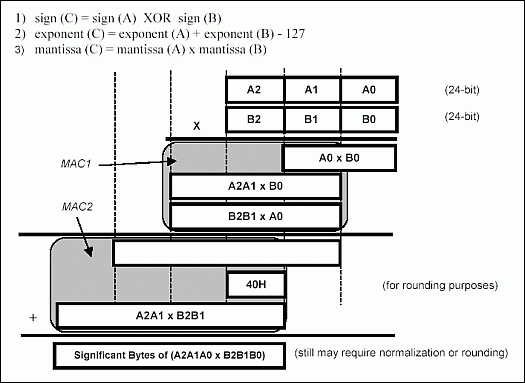### Application Example (continued)-Code Listing

To simplify the example, the application code does not support the following multiplicands or products: 0, -0, Infinity, -Infinity, NaN (not a number), denormalized values. Also, the code performs biased rounding of the product (i.e., if the product falls exactly between two representable numbers, it will be rounded up). This software is available for download.

```;-----------------------------------------------------------
; Single Precision Floating Point Multiply
; (does not support 0, -0, INF, -INF, NaN, denormalized #'s)
;
; inputs: R0-R1-R2-R3 = multiplicand1 (float)
; R4-R5-R6-R7 = multiplicand2 (float)
;
; output: R4-R5-R6-R7 = product (float)
;
; uses: bits - psw.5, c
; SFRs - acc, b, ma, mb, mc, mcnt1
;
;-----------------------------------------------------------
;--------------------- determine sign ----------------------
; [x] = Machine Cycle Count
fmult: mov a, r0 ; 
xrl a, r4 ; 
rlc a ; 
;-------------------- calc new exponent --------------------
mov a, r1 ; 
setb acc.7 ;  assumed 1 before decimal
xch a, r1 ; 
rlc a ;  get lsbit of exponent
mov a, r0 ;  get upper 7 of exp
rlc a ;  exponent byte in acc
mov r0, a ;  store exp to R0
mov a, r5 ; 
setb acc.7 ;  assumed 1 before decimal
xch a, r5 ; 
rlc a ;  get lsbit of exponent
mov a, r4 ;  get upper 7 of exp
rlc a ;  exponent byte in acc
add a, #81h ;  subtract exponent bias
mov r4, a ;  store to r4
;---------------- multiply significands --------------------
orl mcnt1, #10h ;  CLM=1 clear MA, MB, MC
mov a, r7 ; 
mov b, r3 ; 
mul ab ;  (A0 * B0)
mov mc, b ;  msb of (A0*B0) into MC
clr a ;  A=00
mov mb, r3 ;  ----------------------
mov mb, a ;  .
mov ma, r6 ;  .
mov ma, r5 ;  .
nop ;  (B2B1 * A0)
nop ;  .
nop ;  .
nop ;  .
nop ;  .
nop ;  ----------------------
mov mb, r2 ;  ----------------------
mov mb, r1 ;  .
mov ma, r7 ;  .
mov ma, a ;  .
nop ;  (A2A1 * B0)
nop ;  .
nop ;  .
nop ;  .
nop ;  .
nop ;  ----------------------
mov a, mc ;  msbyte MC not needed
push mc ;  save
push mc ;  save
mov a, mc ; 
orl mcnt1, #10h ;  CLM=1 clear MA, MB, MC
mov mc, a ;  reload 40-bit ACC
jnc mc2 ;  carry from add?
pop acc ;  yes.
inc a ;  add carry.
mov mc, a ; 
jnz mc1 ;  carry from add?
pop acc ;  yes.
inc a ;  add carry
mov mc, a ;  40-bit ACC loaded
sjmp mc0 ; 
mc1: pop mc ; 
mc0: mov mb, r2 ;  ---------------------
mov mb, r1 ;  .
mov ma, r6 ;  .
mov ma, r5 ;  .
nop ;  (A2A1 * B2B1)
nop ;  .
nop ;  .
nop ;  .
nop ;  .
nop ;  ---------------------
mov a, mc ;  msbyte MC not needed
mov r5, mc ;  store 3 msbytes
mov r6, mc ; 
mov r7, mc ; 
push mc ;  store for norm or round
mov a, r5 ; 
jnb acc.7, norm ;  need to normalize?
pop acc ;  no
cjne a, #0C0h, rnd ; 
rnd: jc no_rnd ;  need to round?
inc r7 ;  yes
mov a, r7 ; 
jnz no_rnd ;  did we carry?
inc r6 ;  yes
mov a, r6 ; 
jnz no_rnd ;  carry again?
inc r5 ;  yes
no_rnd: inc r4 ;  inc exponent
mov a, r4 ;  get exponent
mov c, psw.5 ;  get sign
rrc a ;  sign -> msbit
mov r4, a ;  store byte in R4
jnc exp0 ;  lsbit of exponent = 1?
ret ;  yes.
norm: pop acc ; 
rlc a ;  rotate msbit -> carry bit
mov a, r7 ;  -------------------------
rlc a ;  .
mov r7, a ;  .
mov a, r6 ;  rotate each byte by 1bit
rlc a ;  using the carry bit
mov r6, a ;  .
mov a, r5 ;  .
rlc a ;  .
mov r5, a ;  .
mov a, r4 ;  -------------------------
mov c, psw.5 ;  get sign
rrc a ;  sign -> msbit
mov r4, a ;  store byte in R4
jc done ;  lsbit of exponent = 1?
exp0: mov a, r5 ;  no.
cpl acc.7 ;  lsbit of exponent = 0.
mov r5, a ; 
done: ret ; 
; -------
; Total cycles min =  @40Mhz = 14.1us
; max =  @40Mhz = 16.8us
```